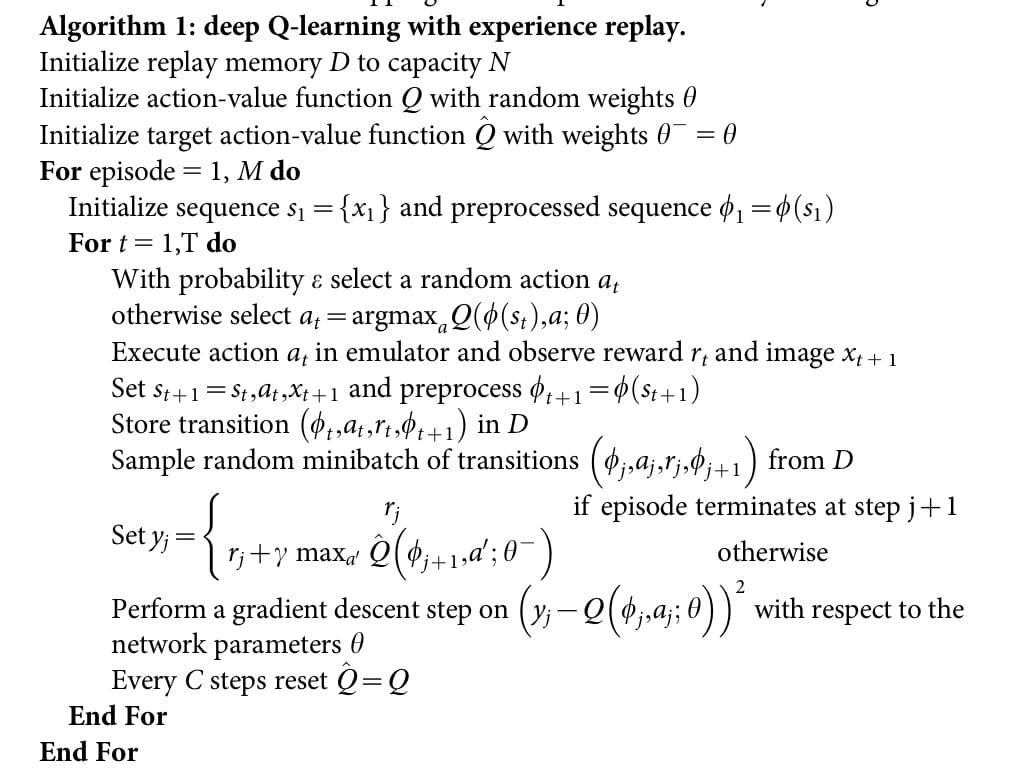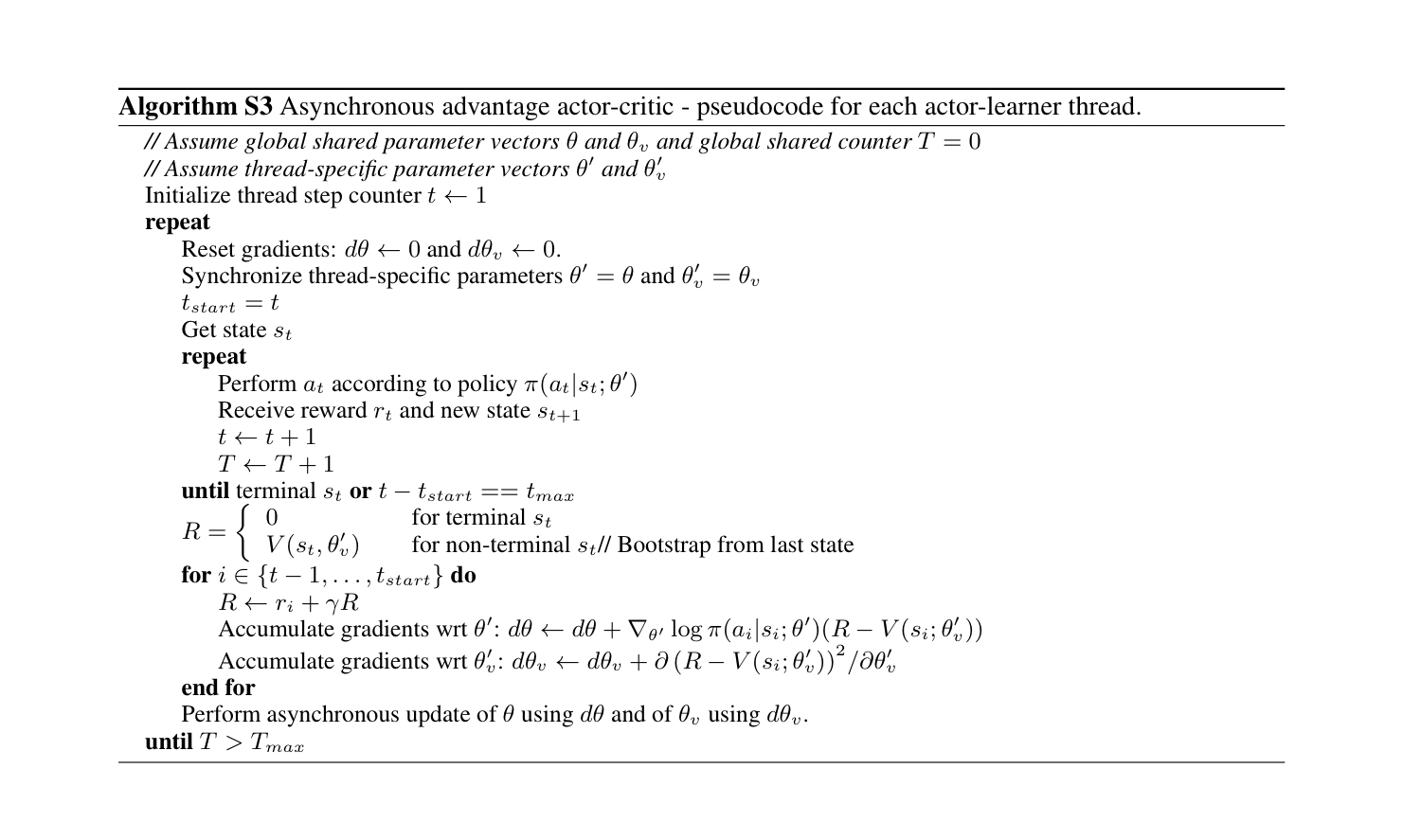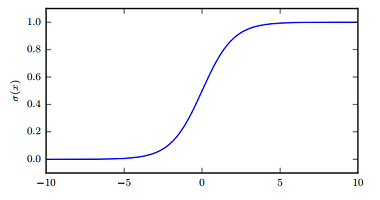# NIUHE

## Deep Q-Network

Deep Q-Network (DQN) 是由DeepMind的Mnih等人于2013年提出的算法，该算法成功把深度学习应用到了RL领域，并（一定程度上）解决了训练不稳定的问题，在玩Atari游戏中取得了非常好的结果。

1. 同一个观测序列中的数据相关性较大
2. 当 Q-value 发生了很小的改变，可能导致整个策略（policy）发生较大变化，从而导致 Q-value 和目标 $r + \gamma * \max_{a'}Q(s' ,a')$ 的差距不稳定

DQN使用了两个trick来解决上述问题：

• Experience replay
• 使用经验池缓存数据，每次训练时从经验池里sample数据，从而降低训练数据之间的相关性
• Two Q networks
• 一个网络用来生成 Q-target，另一个网络进行探索；每隔一定时间两个网络进行同步
• 这样使得 Q-target 相对稳定## Asynchronous Actor Critic

Asynchronous Actor Critic (A3C) 也是由DeepMind的Mnih等人提出的算法，于2016年发表在ICML上。不同于DQN的是，A3C属于策略梯度（Policy Gradient）类算法，而DQN是基于value的；相同的是，A3C也在Atari游戏上取得了非常好的结果（强于DQN）。

1. 使用更多的内存和计算资源
2. 只能使用 off-policy 的RL算法（学习 old policy 产生的数据）

• 并行地使用多个 agent 在各自的环境里探索，每个 agent 在同一时刻探索的内容各不相同，从而降低了数据相关性
• 在CPU上训练更加高效

1. 同步线程专属网络（$\theta', \theta_v'$）和全局网络（$\theta, theta_v$
2. 每个 agent 使用线程专属网络各自进行探索
3. 根据线程专属网络计算梯度：$d\theta, d\theta_v$
4. 使用 $d\theta, d\theta_v$ 更新全局网络（$\theta, theta_v$
5. 回到第一步• 主线程向子线程传参数，子线程向主线程传梯度
• agent 和 critic 共用一个网络，输出分为两头
• 增加了熵正则化（鼓励探索）
• $\triangledown_{\theta'}\log\pi(a_t|s_t;\theta')(R_t-V(s_t;\theta_v))+\beta\triangledown_{\theta'}H(\pi(s_t; \theta'))$
• $H(X) = E[-\log P(X)]$
• 代码参考：https://github.com/NeymarL/Pacman-RL/blob/master/src/a3c.py
• ：计算 policy loss 中的 advantage 的时候不能保留其梯度，否则 policy 的梯度会流入 value network 中，产生bug

$\hat{A}_t^{k}:=\sum_{l=0}^{k-1}\gamma^l\delta_{t+l}^V=-V(s_t)+r_t+\gamma r_{t+1}+...+\gamma^{k-1}r_{t+k-1}+\gamma^kV(s_{t+k})$

$\text{GAE}(\lambda)$ 的定义为这些 $k$ 步估计的指数平均，即 TD($\lambda$) error： \begin{align} \text{GAE}_t(\lambda)&:=(1-\lambda)(\hat{A}_t^{(1)}+\lambda\hat{A}_t^{(2)}+\lambda^2\hat{A}_t^{(3)}+...)\\ &=(1-\lambda)(\delta_t^V+\lambda(\delta_t^V-\gamma\delta_{t+1}^V)+...)\\ &=\sum_{l=0}^\infty(\gamma\lambda)^l\delta_{t+l}^V \end{align} 代码实现

## 其他

### Batch-Normalization

• 梯度截断
• 初始化
• RELU

Inference时 $\mu_B$$\sigma^2_B$ 固定。

• 在模型训练过程中进行白化操作会带来过高的计算代价和运算时间

### Activation Layers

#### ReLU

ReLU 的过程更接近生物神经元的作用过程

Leaky ReLU

ReLU 及其扩展都是基于一个原则，那就是如果它们的行为更接近线性，那么模型更容易优化。 $g(z; \alpha) = \max(0, z) + \alpha \min(0, z)$ $\alpha$ 为固定值或可学习参数。

#### Sigmoid & Tanh$g(z) = \frac{1}{1 + e^{-z}}$

• sigmoid 常作为输出单元用来预测二值型变量取值为 1 的概率
• sigmoid 函数在输入取绝对值非常大的正值或负值时会出现饱和（saturate）现象，在图像上表现为开始变得很平，此时函数会对输入的微小改变会变得不敏感。仅当输入接近 0 时才会变得敏感。从而使得学习变困难。
• 如果要使用 sigmoid 作为激活函数时（浅层网络），tanh 通常要比 sigmoid 函数表现更好。

### Bagging

Bagging（bootstrap aggregating）是通过结合几个模型降低泛化误差的技术 (Breiman, 1994)。